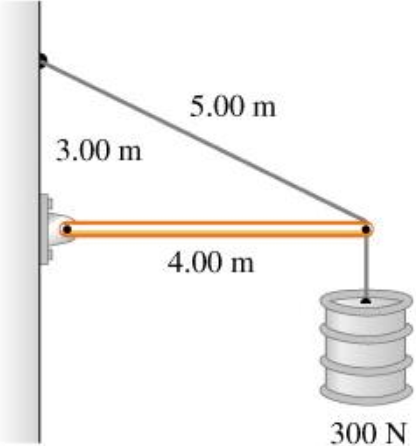# Problem: The horizontal beam in the figure below weighs 200 N, and its center of gravity is at its center.A. Find the tension in the cable (N).B. Find the horizontal component of the force exerted on the beam at the wall (N).C. Find the vertical component of the force exerted on the beam at the wall (N).

###### FREE Expert Solution

A.

Equilibrium condition for torques about the pivot:

$\overline{){\mathbf{\Sigma }}{\mathbf{\tau }}{\mathbf{=}}{\mathbf{0}}}$

(4.00)T sin (θ) - (4.00)(300) - (4.00/2)(200) = 0

sin θ = 3.00/5.00

87% (165 ratings)###### Problem Details

The horizontal beam in the figure below weighs 200 N, and its center of gravity is at its center.

A. Find the tension in the cable (N).

B. Find the horizontal component of the force exerted on the beam at the wall (N).

C. Find the vertical component of the force exerted on the beam at the wall (N).